• 上因为最早由[Fisher, 1936]提出,亦称“Fisher判别分析”。 LDA的基本思想:给定训练样例集,设法将样例投影到一条直线上,使得同类样例的投影点尽可能接近、异类样例的投影点尽可能远离;在对新样本进行分类时,将其投影...
• 多分类核Fisher判别分析法，里面我给了一个例子，用的是鸢尾花数据集，四维三类，降到二维，核函数有高斯核，线性核，多项式核等，需要自取
• ## Fisher判别分析详解

万次阅读 多人点赞 2019-05-16 13:24:56
Fisher判别分析 将高维度空间的样本投影到低维空间上，使得投影后的样本数据在新的子空间上有最小的类内距离以及最大的类间距离，使得在该子空间上有最佳的可分离性 可以看出右侧投影后具有更好的可分离性。 Fisher...
Fisher判别分析
将高维度空间的样本投影到低维空间上，使得投影后的样本数据在新的子空间上有最小的类内距离以及最大的类间距离，使得在该子空间上有最佳的可分离性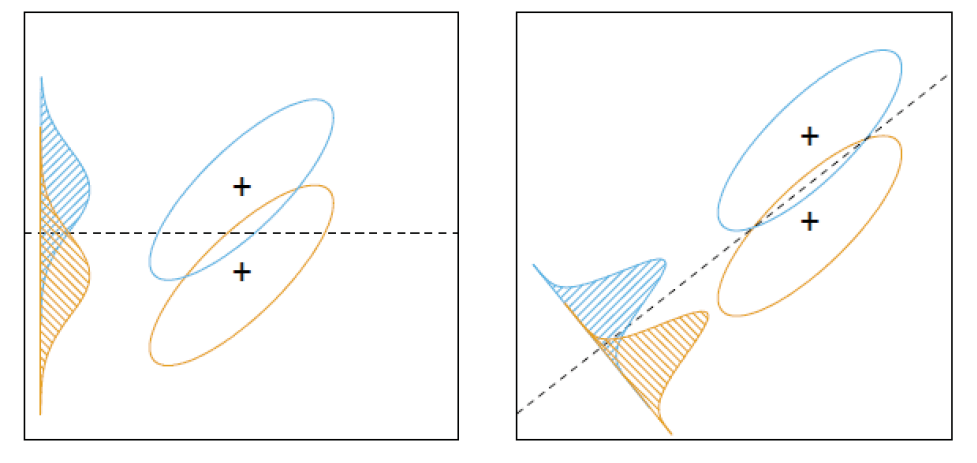可以看出右侧投影后具有更好的可分离性。
Fisher判别分析和PCA差别
刚学完感觉两个很类似，实际上两个方法是从不同的角度来降维。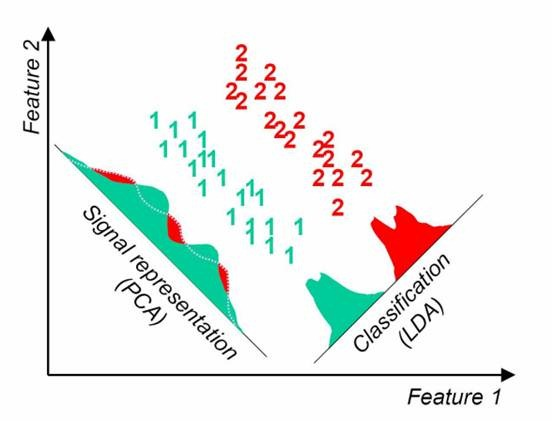PCA是找到方差尽可能大的维度，使得信息尽可能都保存，不考虑样本的可分离性，不具备预测功能。 LAD(线性判别分析) 是找到一个低维的空间，投影后，使得可分离性最佳，投影后可进行判别以及对新的样本进行预测。
Fisher判别详解
Fisher判别分析是要实现有最大的类间距离，以及最小的类内距离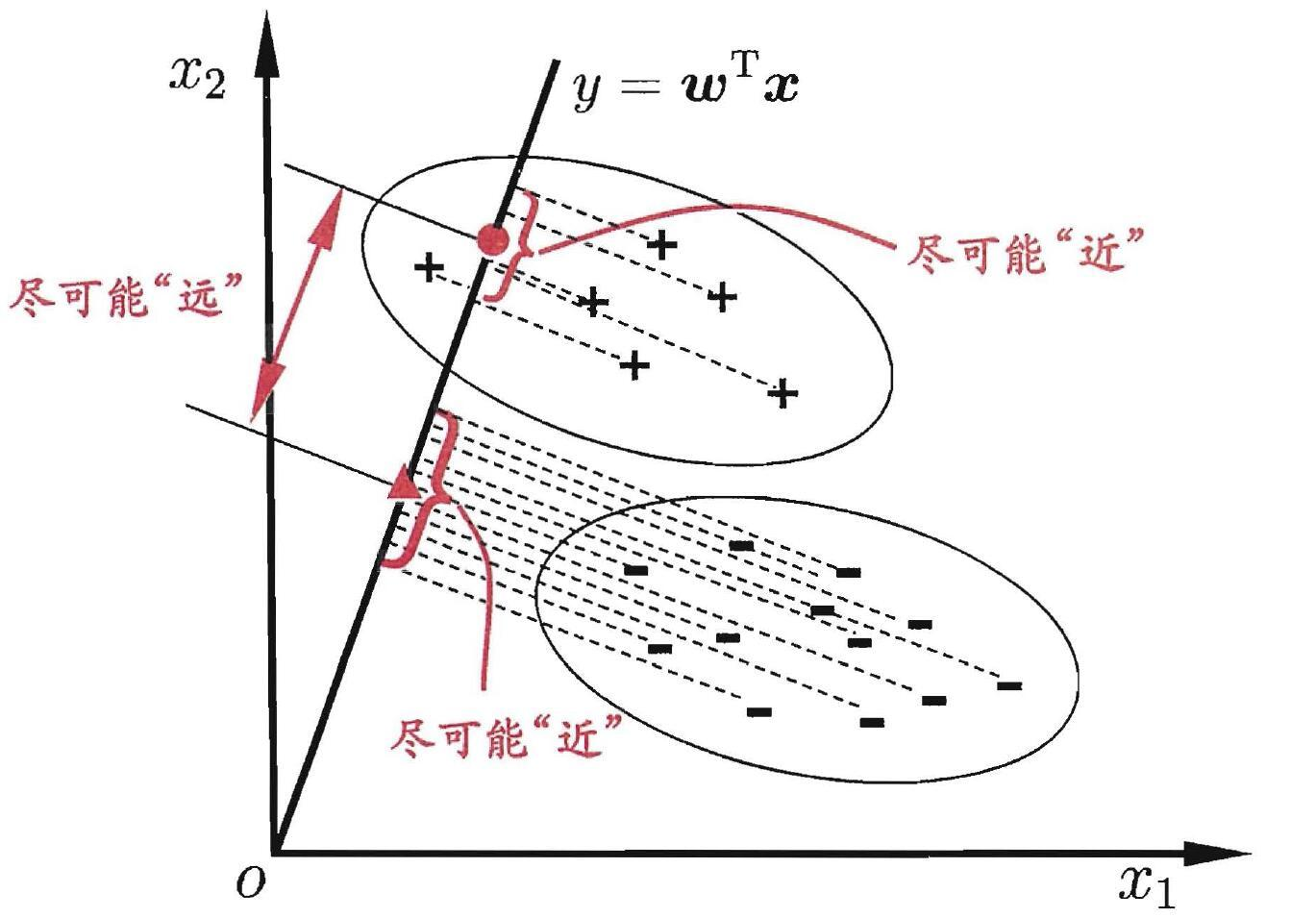用二分类来分析，设样本数据集为

D

=

{

(

X

,

Y

)

}

，

X

i

是

第

i

类

样

本

的

集

合

，

i

∈

{

0

,

1

}

D=\{(X,Y)\}，X_i是第i类样本的集合，i\in\{\ 0,1\}

,要找的最佳投影为

W

W

。
记

μ

i

\mu_i

为第i类样本质心,

N

i

N_i

为第i类的数量

μ

i

=

1

N

i

∑

x

∈

X

i

x

\mu_i=\frac{1}{N_i}\sum_{x\in X_i} x

最大的类间距离 即要投影后两个样本的质心离得越远越好，那么就能得到

J

0

=

(

W

T

μ

1

−

W

T

μ

0

)

2

=

(

W

T

(

μ

1

−

μ

0

)

)

2

=

(

W

T

(

μ

1

−

μ

0

)

)

(

W

T

(

μ

1

−

μ

0

)

)

T

=

W

T

(

μ

1

−

μ

0

)

(

μ

1

−

μ

0

)

T

W

J_0=(W^T\mu_1-W^T\mu0)^2=(W^T(\mu_1-\mu0))^2=(W^T(\mu_1-\mu0))(W^T(\mu_1-\mu0))^T=W^T(\mu_1-\mu0)(\mu_1-\mu0)^TW

S

0

=

(

μ

1

−

μ

0

)

(

μ

1

−

μ

0

)

T

S_0=(\mu_1-\mu0)(\mu_1-\mu0)^T

，那么

J

0

=

W

T

S

0

W

J_0=W^TS_0W

最小的类内距离 即要使得投影后同一类的样本点尽可能聚拢在一起，离质心越近越好。

J

1

=

∑

x

∈

X

i

(

W

T

x

−

W

T

u

i

)

2

=

∑

x

∈

X

i

(

W

T

(

x

−

u

i

)

)

(

W

T

(

x

−

u

i

)

)

T

=

∑

x

∈

X

i

W

T

(

x

−

u

i

)

(

x

−

u

i

)

T

W

J1=\sum_{x\in X_i} (W^Tx-W^Tu_i)^2=\sum_{x\in X_i} (W^T(x-u_i))(W^T(x-u_i))^T=\sum_{x\in X_i} W^T(x-u_i)(x-u_i)^TW

S

1

=

∑

x

∈

X

i

(

x

−

u

i

)

(

x

−

u

i

)

T

S_1=\sum_{x\in X_i}(x-u_i)(x-u_i)^T

,那么

J

1

=

W

T

S

1

W

J1=W^TS_1W

那么我们现在要使得

J

0

J0

尽可能大，

J

1

J_1

尽可能小，那么就是最大化

J

=

J

0

J

1

=

W

T

S

0

W

W

T

S

1

W

J=\frac{J_0}{J_1}=\frac{W^TS_0W}{W^TS_1W}

分式上下都有W，那么W和

α

W

\alpha W

效果是一样的，不妨令

W

T

S

1

W

=

1

W^TS_1W=1

，那么优化条件变成

J

=

W

T

S

0

W

,

s

.

t

.

:

W

T

S

1

W

=

1

J=W^TS_0W,s.t. :W^TS_1W=1

用拉格朗日乘子法求解，

L

(

W

,

λ

)

=

W

T

S

0

W

−

λ

(

W

T

S

1

W

−

1

)

L(W,\lambda)=W^TS_0W-\lambda(W^TS_1W-1)

d

L

=

(

d

w

)

T

S

0

W

+

W

T

S

0

d

W

−

λ

(

(

d

w

)

T

S

1

W

+

W

T

S

1

d

W

)

dL=(dw)^TS_0W+W^TS_0dW-\lambda((dw)^TS_1W+W^TS_1dW)

=

W

T

S

0

T

d

W

+

W

T

S

0

d

W

−

λ

(

W

T

S

1

T

d

W

+

W

T

S

1

d

W

)

=W^TS_0^TdW+W^TS_0dW-\lambda(W^TS_1^TdW+W^TS_1dW)

=

(

2

W

T

S

0

−

2

λ

W

T

S

1

)

d

W

=(2W^TS_0-2\lambda W^TS_1)dW

运算过程是用到矩阵求导,

S

0

T

=

S

0

S_0^T=S_0

。 那么

∂

L

∂

W

=

(

2

W

T

S

0

−

2

λ

W

T

S

1

)

T

=

2

S

0

W

−

2

λ

S

1

W

\frac{\partial L}{\partial W}=(2W^TS_0-2\lambda W^TS_1)^T=2S_0W-2\lambda S_1W

,导数为0处，

S

0

W

=

λ

S

1

W

S_0W=\lambda S_1W

当

S

1

S_1

可逆的时候，

λ

W

=

S

1

−

1

S

0

W

\lambda W=S_1^{-1}S0W

,那么最优的

W

W

即为

S

1

−

1

S

0

S_1^{-1}S_0

的最大的特征向量。 如果要将

X

∈

R

n

X\in R^n

投影到

R

d

(

d

&lt;

n

)

R^d (d&lt;n)

的子空间中，则取前d个特征向量。
注意点:
1.LDA处理后的维度要小于类别数C，Sb的秩肯定小于等于C-1。 2.Sw矩阵不可逆情况很多，可以先用PCA处理成C-1维后再用LDA，效果挺好的 3.多分类情况下Sb用全局离散度矩阵减去类内离散度矩阵
参考博客：
线性分类器之Fisher线性判别 线性判别分析（Linear Discriminant Analysis）（一） 数模系列(7)：Fisher判别分析（Fisher Discriminant Analysis）
展开全文机器学习
• Fisher判别分析法(FDA)应用于硫化矿石自燃倾向性等级分类问题中,选用主要矿物及含量、吸氧速度常数平均值、自热点、自燃点4项指标作为判别因子,建立硫化矿石自燃倾向性等级分类的FDA模型。选取新桥硫铁矿13个矿样...
• Fisher判别分析法在判别混合水时,只能将此确定为最相像的某一分组,从而发生误判,因此在Fisher判别分析理论的基础上引入了质心距评价法,剔除可能为混合水样的样本数据,可以提高判别结果的准确率。为此选取13种水化学...
• Fisher线性判别分析 支持向量机（SVM） 该代码是用Matlab编写的，需要用户进行安装。 包括三个脸部数据库，以在脸部的姿势和照明有变化的情况下测试该算法的有效性。 理论 Fisher线性判别分析 Fisher LDA与主成分...
• Fisher算法python实现含训练集和测试集，Fisher算法也叫Fisher判别分析，或线性判别分析（LDA）
• 应用两总体Fisher判别分析法,选取电磁辐射强度、钻屑瓦斯解吸指标、钻屑量、钻孔瓦斯涌出初速度、软煤厚度为预测指标,提出了基于两总体Fisher判别分析法的煤与瓦斯突出预测模型。以河南能源化工集团有限公司九里山...
• 采用Fisher鉴别分析对mnist数据集当中的手写数字0,1进行识别
• lfda, 局部Fisher判别分析的R 包 lfda软件用于对和visualizing局部Fisher判别分析，核局部Fisher判别分析和半监督局部Fisher判别分析算法进行 package 。这些算法的介绍和应用可以在这里找到，这里是，这里是 。 ...
• 机器学习之Fisher判别分析一、算法描述1、W的确定2、阈值的确定3、Fisher线性判别的决策规则4、群内离散度”（样本类内离散矩阵）、“群间离散度”（总类内离散矩阵）二、Python代码实现 一、算法描述 Fisher线性...


机器学习之Fisher判别分析
一、算法描述1、W的确定2、阈值的确定3、Fisher线性判别的决策规则4、群内离散度”（样本类内离散矩阵）、“群间离散度”（总类内离散矩阵）
二、Python代码实现

一、算法描述
Fisher线性判别分析的基本思想：选择一个投影方向（线性变换，线性组合），将高维问题降低到一维问题来解决，同时变换后的一维数据满足每一类内部的样本尽可能聚集在一起，不同类的样本相隔尽可能地远。 Fisher线性判别分析，就是通过给定的训练数据，确定投影方向W和阈值w0， 即确定线性判别函数，然后根据这个线性判别函数，对测试数据进行测试，得到测试数据的类别。
Fisher判别分析是要实现有最大的类间距离，以及最小的类内距离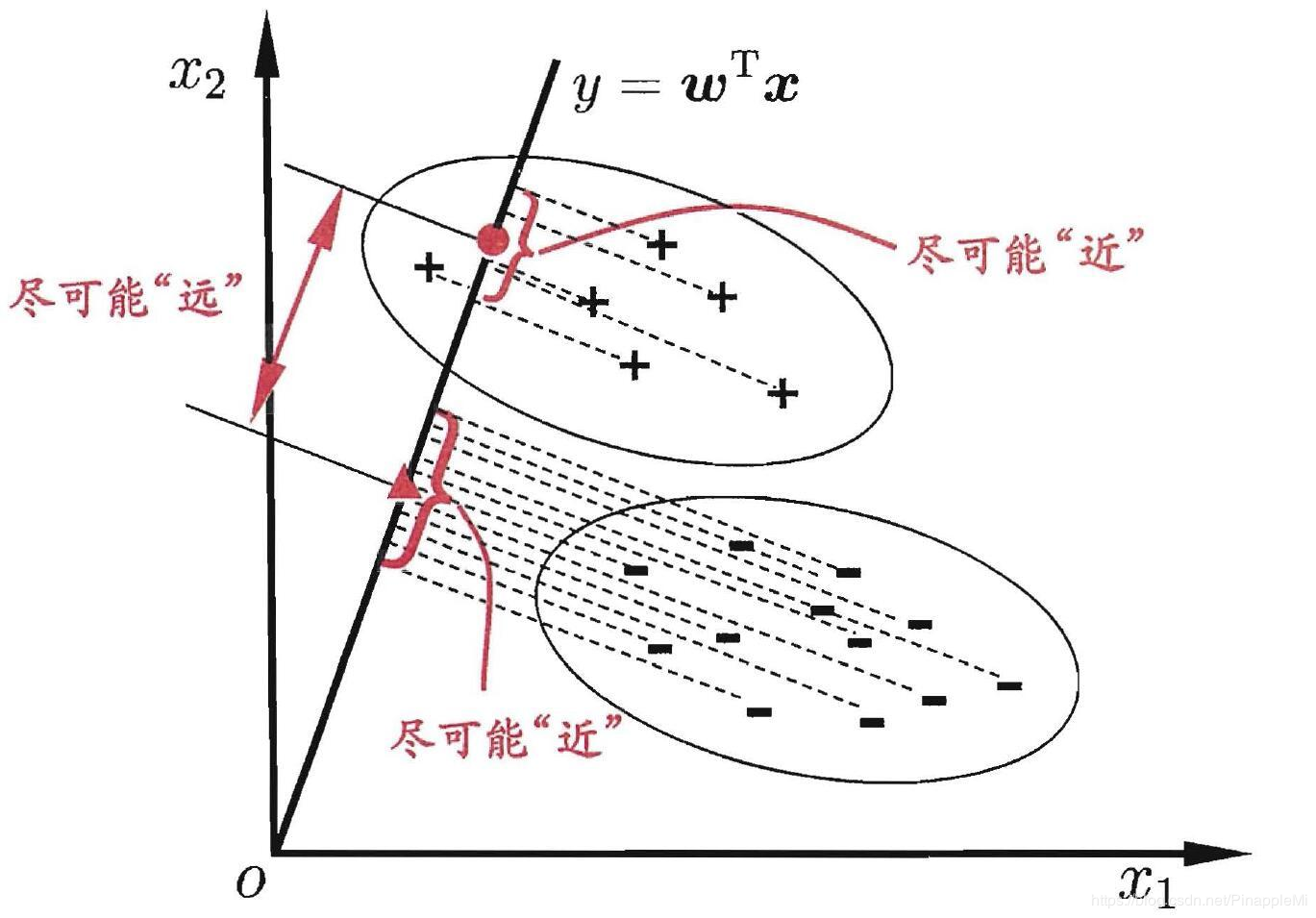线性判别函数的一般形式可表示成：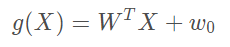其中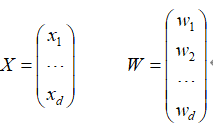Fisher选择投影方向W的原则，即使原样本向量在该方向上的投影能兼顾类间分布尽可能分开，类内样本投影尽可能密集的要求。 如下为具体步骤：
1、W的确定
各类样本均值向量mi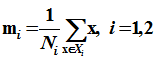样本类内离散度矩阵Si和总类内离散度矩阵Sw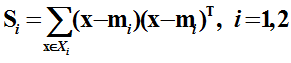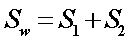样本类间离散度矩阵Sb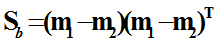在投影后的一维空间中，各类样本均值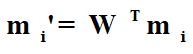样本类内离散度和总类内离散度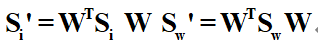样本类间离散度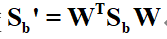Fisher准则函数为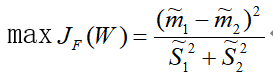2、阈值的确定
W0是个常数，称为阈值权,对于两类问题的线性分类器可以采用下属决策规则: 令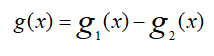则： 如果g(x)>0,则决策x∈W1；如果g(x)<0,则决策x∈W2；如果g(x)=0,则可将x任意分到某一类，或拒绝。
3、Fisher线性判别的决策规则
Fisher准则函数满足两个性质： 1.投影后，各类样本内部尽可能密集，即总类内离散度越小越好。 2.投影后，各类样本尽可能离得远，即样本类间离散度越大越好。
根据这个性质确定准则函数，根据使准则函数取得最大值，可求出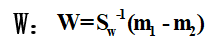这就是Fisher判别准则下的最优投影方向。 最后得到决策规则 若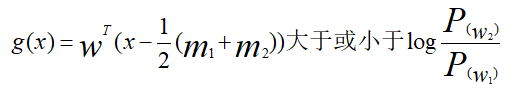则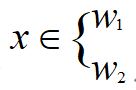对于某一个未知类别的样本向量x，如果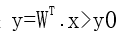x>y0，则x∈w1；否则x∈w2。
4、群内离散度”（样本类内离散矩阵）、“群间离散度”（总类内离散矩阵）
样本在d维特征空间的描述量，根据上面的推导可以发现： 群内离散度”（样本类内离散矩阵）Si的计算公式为：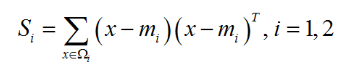群间离散度”（总体类离散度矩阵）Sw的计算公式为：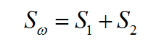二、Python代码实现
import pandas as pd
import numpy as np
import matplotlib.pyplot as plt
path=r'D:\Workspaces\Jupyter-notebook\datasets\mldata\Iris.data'
Iris1=df.values[0:50,0:4]
Iris2=df.values[50:100,0:4]
Iris3=df.values[100:150,0:4]
m1=np.mean(Iris1,axis=0)
m2=np.mean(Iris2,axis=0)
m3=np.mean(Iris3,axis=0)
s1=np.zeros((4,4))
s2=np.zeros((4,4))
s3=np.zeros((4,4))
for i in range(0,30,1):
a=Iris1[i,:]-m1
a=np.array([a])
b=a.T
s1=s1+np.dot(b,a)
for i in range(0,30,1):
c=Iris2[i,:]-m2
c=np.array([c])
d=c.T
s2=s2+np.dot(d,c)
#s2=s2+np.dot((Iris2[i,:]-m2).T,(Iris2[i,:]-m2))
for i in range(0,30,1):
a=Iris3[i,:]-m3
a=np.array([a])
b=a.T
s3=s3+np.dot(b,a)
sw12=s1+s2
sw13=s1+s3
sw23=s2+s3
#投影方向
a=np.array([m1-m2])
sw12=np.array(sw12,dtype='float')
sw13=np.array(sw13,dtype='float')
sw23=np.array(sw23,dtype='float')
#判别函数以及T
#需要先将m1-m2转化成矩阵才能进行求其转置矩阵
a=m1-m2
a=np.array([a])
a=a.T
b=m1-m3
b=np.array([b])
b=b.T
c=m2-m3
c=np.array([c])
c=c.T
w12=(np.dot(np.linalg.inv(sw12),a)).T
w13=(np.dot(np.linalg.inv(sw13),b)).T
w23=(np.dot(np.linalg.inv(sw23),c)).T
#print(m1+m2) #1x4维度  invsw12 4x4维度  m1-m2 4x1维度
T12=-0.5*(np.dot(np.dot((m1+m2),np.linalg.inv(sw12)),a))
T13=-0.5*(np.dot(np.dot((m1+m3),np.linalg.inv(sw13)),b))
T23=-0.5*(np.dot(np.dot((m2+m3),np.linalg.inv(sw23)),c))
kind1=0
kind2=0
kind3=0
newiris1=[]
newiris2=[]
newiris3=[]
for i in range(29,49):
x=Iris1[i,:]
x=np.array([x])
g12=np.dot(w12,x.T)+T12
g13=np.dot(w13,x.T)+T13
g23=np.dot(w23,x.T)+T23
if g12>0 and g13>0:
newiris1.extend(x)
kind1=kind1+1
elif g12<0 and g23>0:
newiris2.extend(x)
elif g13<0 and g23<0 :
newiris3.extend(x)
#print(newiris1)
for i in range(29,49):
x=Iris2[i,:]
x=np.array([x])
g12=np.dot(w12,x.T)+T12
g13=np.dot(w13,x.T)+T13
g23=np.dot(w23,x.T)+T23
if g12>0 and g13>0:
newiris1.extend(x)
elif g12<0 and g23>0:

newiris2.extend(x)
kind2=kind2+1
elif g13<0 and g23<0 :
newiris3.extend(x)
for i in range(29,49):
x=Iris3[i,:]
x=np.array([x])
g12=np.dot(w12,x.T)+T12
g13=np.dot(w13,x.T)+T13
g23=np.dot(w23,x.T)+T23
if g12>0 and g13>0:
newiris1.extend(x)
elif g12<0 and g23>0:
newiris2.extend(x)
elif g13<0 and g23<0 :
newiris3.extend(x)
kind3=kind3+1
#花瓣与花萼的长度散点图
plt.scatter(df.values[:50, 3], df.values[:50, 1], color='red', marker='o', label='setosa')
plt.scatter(df.values[50:100, 3], df.values[50: 100, 1], color='blue', marker='x', label='versicolor')
plt.scatter(df.values[100:150, 3], df.values[100: 150, 1], color='green', label='virginica')
plt.xlabel('petal length')
plt.ylabel('sepal length')
plt.title("花瓣与花萼长度的散点图")
plt.rcParams['font.sans-serif']=['SimHei'] #显示中文标签
plt.rcParams['axes.unicode_minus']=False
plt.legend(loc='upper left')
plt.show()

#花瓣与花萼的宽度度散点图
plt.scatter(df.values[:50, 4], df.values[:50, 2], color='red', marker='o', label='setosa')
plt.scatter(df.values[50:100, 4], df.values[50: 100, 2], color='blue', marker='x', label='versicolor')
plt.scatter(df.values[100:150, 4], df.values[100: 150, 2], color='green', label='virginica')
plt.xlabel('petal width')
plt.ylabel('sepal width')
plt.title("花瓣与花萼宽度的散点图")
plt.legend(loc='upper left')
plt.show()

correct=(kind1+kind2+kind3)/60
print("样本类内离散度矩阵S1：",s1,'\n')
print("样本类内离散度矩阵S2：",s2,'\n')
print("样本类内离散度矩阵S3：",s3,'\n')
print('-----------------------------------------------------------------------------------------------')
print("总体类内离散度矩阵Sw12：",sw12,'\n')
print("总体类内离散度矩阵Sw13：",sw13,'\n')
print("总体类内离散度矩阵Sw23：",sw23,'\n')
print('-----------------------------------------------------------------------------------------------')
print('判断出来的综合正确率：',correct*100,'%')

参考文献 参考地址1 参考地址2
展开全文python 机器学习 人工智能
• 本文基于增强型Fisher判别准则（EFDC），提出了一种新的特征提取方法，称为零空间多样性Fisher判别分析（NSDFDA），用于人脸识别。 提出了基于新优化准则的NSDFDA，这意味着可以在类内散点的零空间中计算所有判别...
• 本文在回顾传统Fisher判别分析理论的基础上,指出了量纲及量纲标准化过程对判别分析过程产生的影响,并建立了一种相对Fisher判别分析方法:。先通过预处理的方法:消除量纲差异带来的虚假影响,然后根据系统要求对观测...
• Fisher判别分析 将高维度空间的样本投影到低维空间上，使得投影后的样本数据在新的子空间上有最小的类内距离以及最大的类间距离，使得在该子空间上有最佳的可分离性 可以看出右侧投影后具有更好的可分离性。 ...
Fisher判别分析
将高维度空间的样本投影到低维空间上，使得投影后的样本数据在新的子空间上有最小的类内距离以及最大的类间距离，使得在该子空间上有最佳的可分离性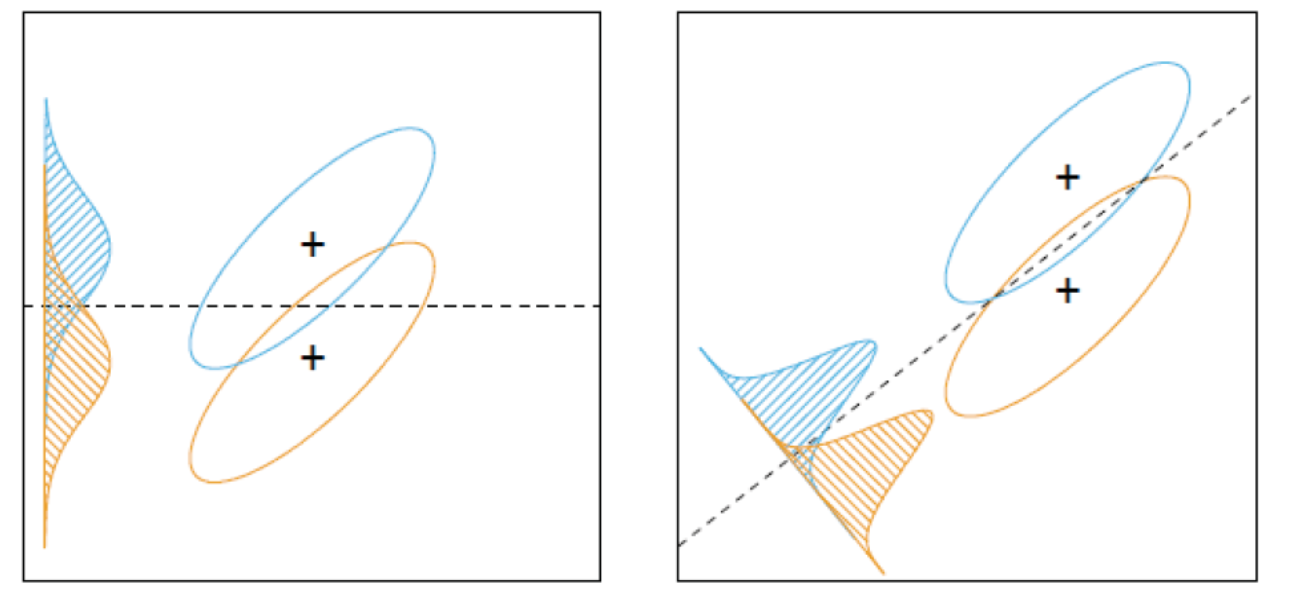可以看出右侧投影后具有更好的可分离性。
Fisher判别分析和PCA差别
刚学完感觉两个很类似，实际上两个方法是从不同的角度来降维。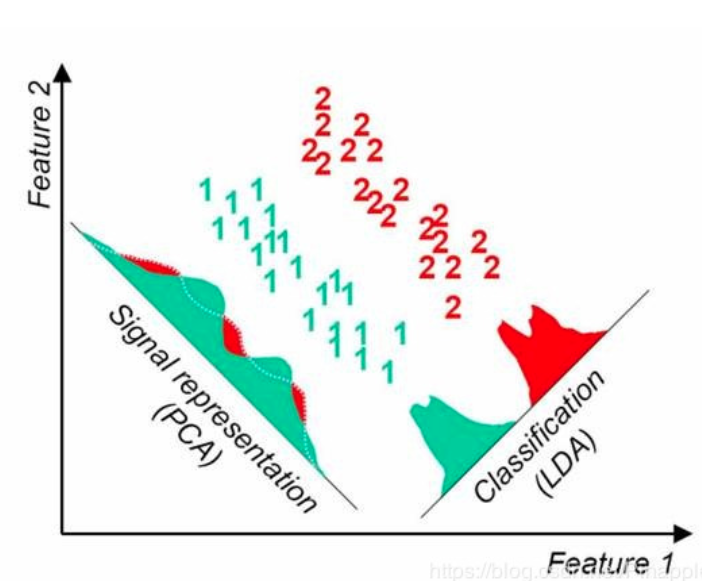Fisher判别详解 Fisher判别分析是要实现有最大的类间距离，以及最小的类内距离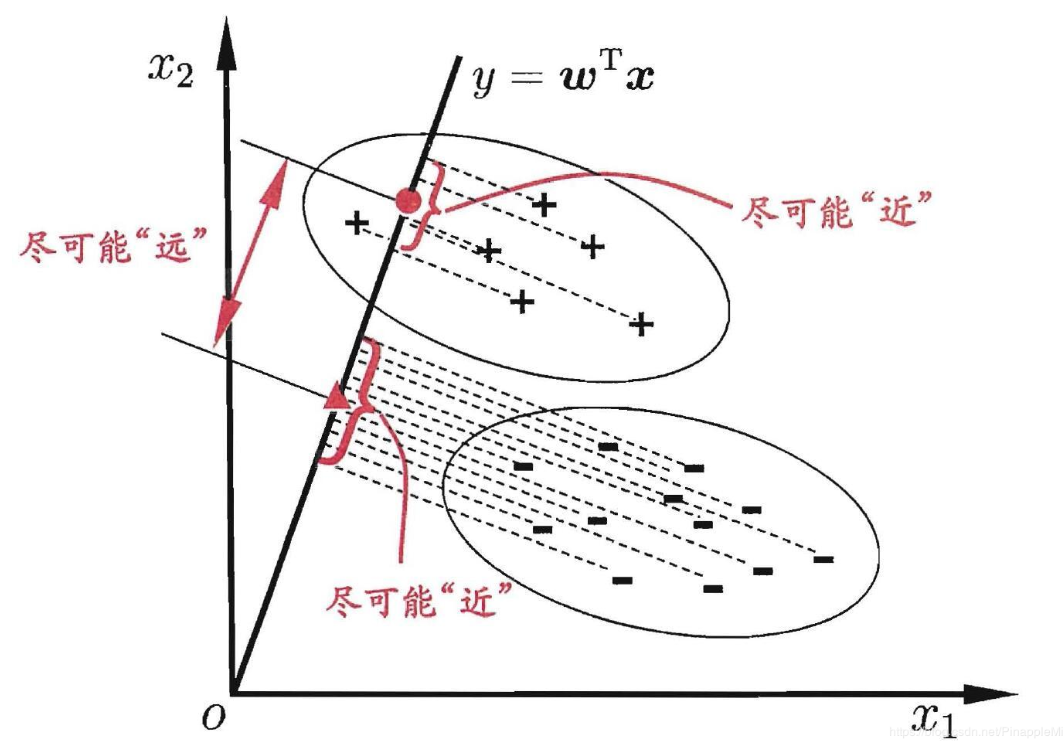注意点:
1.LDA处理后的维度要小于类别数C，Sb的秩肯定小于等于C-1。 2.Sw矩阵不可逆情况很多，可以先用PCA处理成C-1维后再用LDA，效果挺好的 3.多分类情况下Sb用全局离散度矩阵减去类内离散度矩阵

其它详解见：https://blog.csdn.net/PinappleMi/article/details/90261680

展开全文计算机视觉
• 基于半监督局部Fisher判别分析的高光谱图像稀疏度降低研究论文
• 如果需要代码请与我联系，这里只是一些理论数学公式对于写论文有一定的帮助。
• 针对隧道围岩分类问题,基于Fisher判别分析理论,选用岩石质量指标、完整性指数、饱和单轴抗压强度、纵波波速、弹性抗力系数和结构面摩擦因数等6个指标作为判别因子,以30组隧洞围岩数据作为学习样本进行训练,建立相应...
• 提出一种非线性分类方法--基于非线性映射的Fisher判别分析(NM-PDA).首先提取基向量;然后采用Nystrom方法,以基向量为训练样本,将形式未知的非线性映射近似表达为已知形式的非线性映射,这种近似的非线性映射将变量由非...
• fisher判别法是去找一个过原点的直线，这个直线要达到的效果如图： 具体实现方法： 注：这里的Sw为离差阵，也叫做协方差矩阵。 w即为这条直线的方向 直线为Y = wX 临界点y0 = (n0 * u0 + n1 * u1) / (n0 + n1) ...
原理
fisher判别法是去找一个过原点的直线，这个直线要达到的效果如图：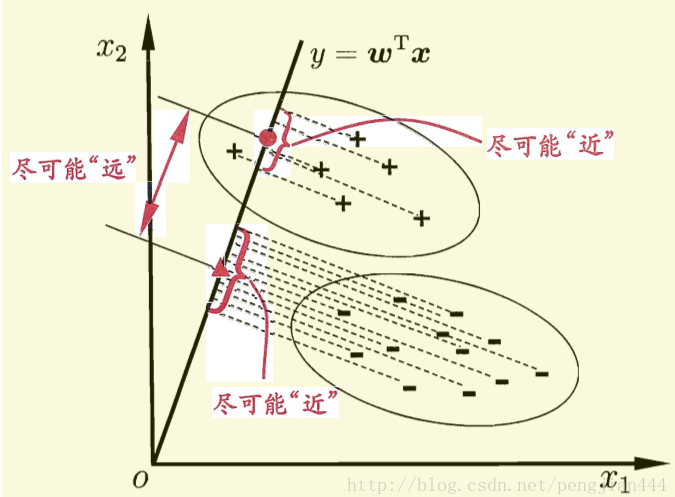具体实现方法：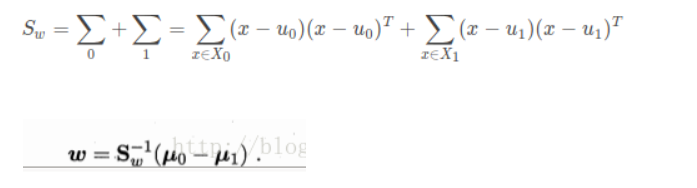注：这里的Sw为离差阵，也叫做协方差矩阵。 w即为这条直线的方向
直线为Y = wX
临界点y0 = (n0 * u0 + n1 * u1) / (n0 + n1)
判别时计算数据在线上的投影，将此投影与聚类的中心投影相比较得出此数据应属于哪一类。
实战
例：为了解某河段As，Pb污染状况，设在甲，乙两地监测，采样测的这两种元素在水中和底泥中的浓度（如下表）。依据这些数据判别未知样本是从哪个区域采得的。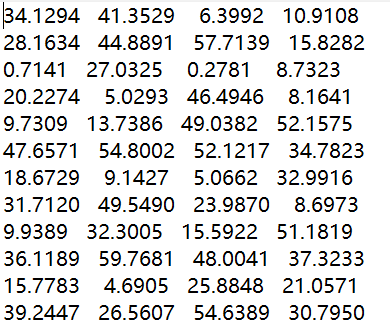注：后两行为需要判别属于哪一类的样本。

clc
clear
x1=X(1:5,:);%甲地的已知数据
x2=X(6:10,:);%乙地的已知数据
r1=size(x1,1);%相当于求甲地的样本数
r2=size(x2,1);%相当于求乙地的样本数
sample=X(11:12,:);%待分类的数据
r3=size(sample,1);
%求各类别的均值
ave1=mean(x1);
avel=ave1';
ave2=mean(x2);
ave2=ave2';
%离差矩阵即协方差矩阵。
s1=cov(x1)*(r1-1);
s2=cov(x2)*(r2-1);
sw=s1+s2;%求出协方差矩阵
w=inv(sw)*(ave1-ave2)*(r1+r2-2);%求出w
y1=mean(w'.*ave1);
y2=mean(w'.*ave2);
y0=(r1*y1+r2*y2)/(r1+r2);
y0=y0';
%利用0,1来区分类别
for i=1:r3
y(i,:)=w'*sample(1,:)';
if y(i,:)>y0
m(i)=0;
else
m(i)=1;
end
end
m %m表示判断结果

展开全文• 为了提高煤炭自燃危险性预测精度,提出了基于KPCA-Fisher判别分析的煤炭自燃预测模型。利用核主成分分析法(KPCA)对相关程度较高的特征指标进行非线性特征提取,将提取出的主成分作为Fisher判别模型的判别因子。选取...
• 为了实时掌握生产过程运行状态, 提出一种基于Fisher 判别分析(FDA) 的过程运行状态在线评价方法. 提出 离线数据分类与识别算法, 以识别不同稳定运行状态的建模数据及其对应的状态等级; 利用FDA提取各个稳定运行 状态...
• 首先得知道Fisher线性判别函数，在处理数据的时候，我们经常遇到高维数据，这个时候往往就会遇到“维数灾难”的问题，即在低维空间可行，那么在高维空间往往却不可行，那么此时我们就可以降数据降维...
• 基于Fisher判别理论建立了急倾斜煤层顶煤可放性分类的Fisher判别分析模型.选取老顶、直接顶、底板、瓦斯、采深、倾角、煤层厚度、坚固性系数、夹矸等9项指标作为Fisher判别分析模型的分类判别指标.利用国内矿区急...
• 很好的fisher判别的代码，简单易懂，明确的解释了该算法的原理，对于接触该类的算法有很好的启发。
• 提出了用于处理多峰问题的本地Fisher判别分析（LFDA）。 它不仅结合了用于保存高维数据局部结构的局部保留投影（LPP）的思想，而且还结合了用于获得判别力的Fisher判别分析（FDA）的思想。 但是，LFDA还存在采样不足...研究论文...